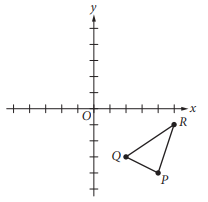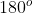Scan QR code or get instant email to install app

Question:

# Triangle PQR lies in the xy-plane, and the coordinates of vertex Q are (2, –3). Triangle PQR is rotated 180° clockwise about the origin and then reflected across the y-axis to produce triangle P′Q′R′, where vertex Q′ corresponds to vertex Q of triangle PQR. What are the coordinates of Q′?A (2, 3)
explanation

1212When triangle PQR is rotatedclockwise about the origin (0, 0), point Q is translated from (2, −3) to (−2, 3) in the xy-plane. Then, after the triangle is reflected, or flipped, across the y-axis, point Q is translated from (−2, 3) to (2, 3).